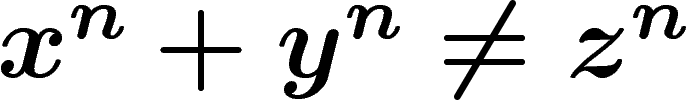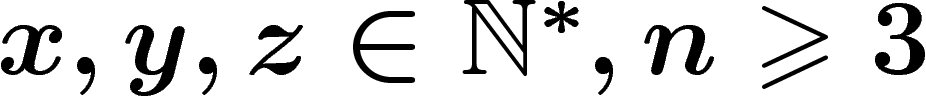# Mathematical programs

Here are a few programs I wrote. All of them are free. The html files are the presentation and documentation, and the archive files are the programs themselves.

Mathematical expression parser in C++: html tar.gz
• Easy-to-use library to interpret mathematical expressions written by the user
• Intended to be as loose as possible when interpreting the user's formulae (like e.g. sinxcos(x)^2)
• Internals use a stack mechanism to store the expressions, so that evaluation is quick

Eqdiff: interactive drawing of vector fields, differential equations, level curves...: html tar.gz zip
• Contains a nice grapher for ordinary functions
• Draws the integral curves of differential equations or vector fields, and level curves
• Gives an overview of the field of integral curves in a single click
• Graphical and easy-to-use
• Nice format for formula input
• Output to standard image formats
• For Unix or Windows

Hypertex: Web page writing system: html
•formulas can be directly inserted into Web pages:for.
• Simple macro processing
• For Unix (C source)

Spectral gap of a graph: html tar.gz
This little code computes an evaluation of the first eigenvalue of the discrete Laplacian of a graph (which, in particular, controls the rate of convergence of the random walk).
• For Unix
• Mathematical reminder included
• Outputs proven upper and lower bounds

Cartographical mappings program: zip
In connection with a mathematical article.
• Lots of projections, with explanations about their construction and mathematical properties
• Allows the user to define its own projections
• For Windows only, and in French

Non-linear fits: html tar.gz zip
A very simple and bad-working program for non-linear fits (polynomial, exponential, logarithmic, power, sinusoidal regressions) I wrote in the 90's.

## Programming reference

LaTeX

C/C++/STL

HTML/CSS/PHP

Perl

Postscript

Back to Main Page